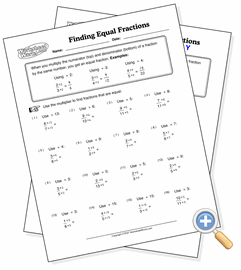# Finding Equal FractionsUse multiplication to create equal fractions

This worksheet provides practice in creating equal fractions using a multiplier. This is an important skill when moving into fraction addition and subtraction. The multiplier is given for each problem and the student must find the equal fraction.